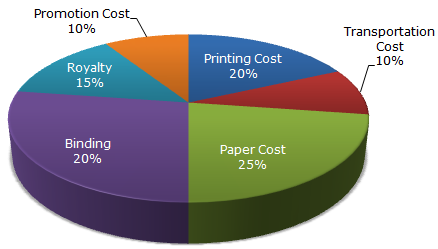# Data Interpretation - Pie Charts - Discussion

The following pie-chart shows the percentage distribution of the expenditure incurred in publishing a book. Study the pie-chart and the answer the questions based on it.

Various Expenditures (in percentage) Incurred in Publishing a Book2.

What is the central angle of the sector corresponding to the expenditure incurred on Royalty?

 [A]. 15° [B]. 24° [C]. 54° [D]. 48°

Explanation:

Central angle corresponding to Royalty = (15% of 360)°
 =15 x 360° 100
= 54°.

 Ankit said: (Mar 22, 2011) You can also findout the answer in this way-: 15*18/5=54 Note:- If we want to convert percentage into degree then we will multiply by 18/5 and if we want to convert degree into percentage then we will multiply by 5/18.

 Areen Sharma said: (May 13, 2011) 15% is the royalty as given in the ques. 10% = 36 degree, 1% = 3.6 degree there for 5% = 18 degree 10+5 = 36+18 15% = 54 simple.

 Kausik said: (Jun 27, 2011) Would anyone please make understand what exactly the question has been asked? 'central angle of the sector' what does it mean? please help me.

 Archana said: (Jul 5, 2011) Central angle means degree, total angle any circle is 360" degree that we measure using protractor.

 Archana said: (Jul 5, 2011) Central angle means degree, total angle any circle is 360" degree that we measure using protractor.

 Namrata said: (Aug 26, 2012) But its given corresponding sector. So we should have taken 15% of either 10% or 20% na?

 Priyanshu Chatturvedi said: (Aug 14, 2014) You can also find out the answer in this way: 15*3.6 = 54. NOTE-[1%=3.6].

 Poonam said: (Oct 22, 2014) 15/100 = 0.15. 360*0.15 = 54.

 Anant said: (Aug 19, 2015) Why central angle correspond to the royalty?

 Jitendra Bharti said: (Oct 4, 2016) If 15% of 360 than 10% is 36. Hence 5% is 18 now 15% is 54.

 King said: (Dec 7, 2016) All the ways are helpful and thank you for the solution.

 Mandar said: (Dec 16, 2016) Now I understand the answer. Thanks for all your explanation.

 Rahul Santra said: (Jan 23, 2017) Here, How we get 360-degree?

 Bhavana said: (May 9, 2017) The total angle in a circle 360 degrees. Royalty occupies 15% of the total. Thus the angle made by royalty is (15/100) *360 =54 degrees.

 Vaidehi said: (Jul 16, 2017) I want to know the trick how to convert degree into a percentage? Please explain.

 Anonymous said: (Sep 23, 2017) Percentage to degree ( X * 18/5). Ex- 15%= ?degree 15 * 18/5= 54degree Degree to percentage ( X * 5/18) Ex- 54degree =?% 54 * 5/18= 15%.

 Sadaf said: (Mar 2, 2018) How to make percent into degree?

 Ladva Dharmesh said: (Mar 17, 2018) If any cost, expenditure can be found in pie chart only multiple 360 can find cost, expenditure.

 Sushmitha said: (Apr 26, 2018) Why we take 15% of 360 can anyone explain me?

 Axel said: (Apr 30, 2018) One circle is equal to 360 angles.

 Vishakha said: (Jul 13, 2018) How 1% =3.6, It's not given 10% is 36. So, how it could be?

 Yeshey Wangchuk said: (Jul 21, 2018) 1% of 360°, 1÷ 100*360= 3.6°. So, 10% means 10÷100* 360. = 3.6°

 Sonam Penjor said: (Aug 2, 2018) How comes 100 here? Please explain.

 Satyananda said: (Sep 18, 2019) Thanks all.

 Akshatha said: (Jan 21, 2020) If royalty=15% , promotion cost=10%. Then total=15+10=25%. For 25% encloses 90 degrees. For 15% encloses how much degrees? So, therefore( 90/25)*15=54°.

 Priyanka said: (Aug 9, 2020) Percent to degree. 360/100=18/5.

 Hiralal Meher said: (Mar 24, 2021) Thank you all for explaining this.

 Ramya said: (Apr 9, 2021) Thankyou all for explaining this.